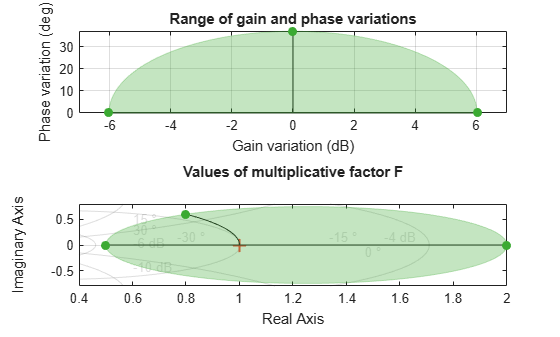## Generate Samples of Uncertain Systems

Use the `usample` function to randomly sample an uncertain model, returning one or more non-uncertain instances of the uncertain model.

### Generating One Sample

If `A` is an uncertain object, then `usample(A)` generates a single sample of `A`.

For example, a sample of a `ureal` is a scalar `double`.

```A = ureal('A',6); B = usample(A) B = 5.7298 ```

Create a 1-by-3 `umat` with `A` and an uncertain complex parameter `C`. A single sample of this `umat` is a 1-by-3 double.

```C = ucomplex('C',2+6j); M = [A C A*A]; usample(M) ans = 5.9785 1.4375 + 6.0290i 35.7428 ```

### Generating Many Samples

If `A` is an uncertain object, then `usample(A,N)` generates `N` samples of `A`.

For example, 20 samples of a `ureal` gives a 1-by-1-20 `double` array.

```B = usample(A,20); size(B) ans = 1 1 20 ```

Similarly, 30 samples of the 1-by-3 `umat` `M` yields a 1-by-3-by-30 array.

```size(usample(M,30)) ans = 1 3 30 ```

See Sample Uncertain Elements to Create Arrays for more information on sampling uncertain objects.

### Sampling Uncertain LTI Dynamics

When sampling an `ultidyn` element or an uncertain object that contains a `ultidyn` element, the result is always a state-space (`ss`) object. The property `SampleStateDimension` of the `ultidyn` class determines the state dimension of the samples. The same is true when sampling `umargin` objects, since these are a type of dynamic uncertainty.

Create a 1-by-1, gain bounded `ultidyn` object with gain bound 4. Verify that the default state dimension for samples is 3.

```del = ultidyn('del',[1 1],'Bound',4); del.SampleStateDimension```
```ans = 3 ```

Sample the uncertain element at 30 points. Verify that this creates a 30-by-1 `ss` array of 1-input, 1-output, 1-state systems.

```rng(0) % for reproducibility delS = usample(del,30); size(delS)```
```30x1 array of state-space models. Each model has 1 outputs, 1 inputs, and 3 states. ```

Plot the Nyquist plot of these samples and add a disk of radius 4, the gain bound of `del`.

```nyquist(delS) hold on; theta = linspace(-pi,pi); plot(del.Bound*exp(sqrt(-1)*theta),'r'); hold off;```Change `SampleStateDimension` to 1, and repeat entire procedure. The Nyquist plots again satisfy the gain bound, but the Nyquist plots are all circles, indicative of 1st order systems.

```del.SampleStateDimension = 1; delS = usample(del,30); nyquist(delS) hold on; theta = linspace(-pi,pi); plot(del.Bound*exp(sqrt(-1)*theta),'r'); hold off;```With `SampleStateDimension `= 1, all Nyquist plots touch the gain boundary at either (–1,0) or (1,0) (frequency = 0 or `Inf`). Higher sampling dimension yields a Nyquist curve that reaches the gain bound at more frequencies, yielding more thorough coverage.

Create a `umargin` object using the default `SampleStateDimension`. The `umargin` block models uncertain gain and phase. The modeled variations are within bounded ranges. For this example use a `umargin` block that captures relative gain variations of a factor of two in either direction, and phase variations of ±30°.

```DGM = getDGM(2,30,'tight'); F = umargin('F')```
```F = Uncertain gain/phase "F" with relative gain change in [0.5,2] and phase change of ±36.9 degrees. ```

The samples of a `umargin` block are also state-space models.

```Fs = usample(F,30); size(Fs)```
```30x1 array of state-space models. Each model has 1 outputs, 1 inputs, and 3 states. ```

Plot the samples on the Nyquist plane.

`nyquist(Fs)`The Nyquist plot of any sample of `F` stays within the disk of uncertainty modeled by `F`. To confirm this bound, use `plot` to examine the uncertainty disk. Compare the Nyquist plot above with the right side of the following plot.

`plot(F)`For further details about the gain and phase uncertainty model, see `umargin`.